## An electric pump rated at 1000W, takes 16 seconds to pump 100 kg of water out to a tank at a height of 10 m. what is the efficiency of the m

Question

An electric pump rated at 1000W, takes 16 seconds to pump 100 kg of water out to a tank at a height of 10 m. what is the efficiency of the motor?​

in progress 0
3 months 2021-08-03T17:10:55+00:00 1 Answers 4 views 0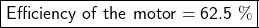Given:

Input Power (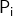)= 1000 W

Mass (m) = 100 kg

Height (h) = 10 m

Time (t) = 16 s

To Find:

Efficiency of the motor

Explanation: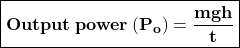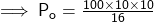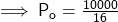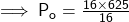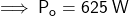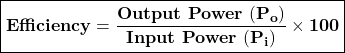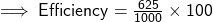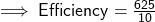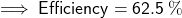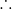Efficiency of the motor = 62.5 %